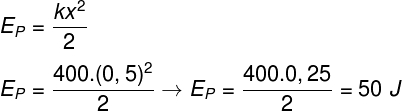Mechanics

# Elastic potential energy

Elastic potential energy is the form of energy that is acquired by elastic bodies capable of returning to their original shape after undergoing some kind of deformation. The elastic potential energy depends on the elastic constant of the body , as well as the size of the deformation suffered by it.

When we apply force to elastic bodies, they deform, starting to store elastic potential energy. If the force ceases, the elastic potential energy will be converted into kinetic energy . In this case, we say that work has been done by the elastic force .

There are several everyday objects that are capable of storing elastic potential energy, check out some of them:

• Springs : some vehicle shock absorbers, mattress springs, etc.;
• Latex : rubber straps, trampoline, rubber ball, etc.

Whether compressed or stretched, elastic bodies will store elastic potential energy. Such energy arises when these bodies do not assume different sizes than they would have if they were not under the action of any force.

When we compress the spring, it gains elastic potential energy and produces a force contrary to compression.

### applications

Most applications of elastic potential energy concern the storage or conversion of kinetic energy by elastic objects . A good example of this is vehicle shock absorbers and bumpers.

The bumpers are made of materials that deform when a collision occurs. In this way, the kinetic energy of the vehicle can be absorbed, reducing the effects on vehicle passengers.

The bumpers are elastic and therefore are able to transform kinetic energy into elastic energy.

## Formula

The formula used to calculate the elastic potential energy of a body relates its elastic constant (k) to the deformation suffered by the body (x), and is shown below, check it out: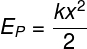p — Elastic potential energy (J – Joules)
k — Elastic constant (N/m)
x — Deformation of the object (m)

Let’s analyze the formula and each of its variables:

• And p : is the measure of the amount of energy that is stored by some elastic body that is under the effect of a force that deforms it, this energy is measured in Joules ;
• k: is a property of each material. Materials that have a high elastic constant are generally harder, as they need to receive a great intensity of force to undergo some deformation. Materials with low elastic constants, in turn, are easily deformed. The spring constant is expressed in Newton per meter (N/m).
• x: is the measure of the deformation suffered by the body. For example, if a spring initially had a size of 20 cm and is stretched to 25 cm, then its deformation will be 5 cm. The unit of measurement for deformation x, however, must always be the meter (m). Therefore, if we were to do a calculation with the deformation of 5 cm, we should use the value of 0.05 m. In addition, it is important to remember that, if the body has its length increased, x will have a positive sign, however, if its length is decreased, the sign of x must be negative.

## Deduction

Elastic potential energy is deduced based on Hooke’s law , which measures the intensity of the elastic force produced by deformed bodies, along with the physical definition of mechanical work :

Hooke’s law states that elastic force arises in reaction to compression or tensioning of elastic bodies. It is a reaction force that always appears in the same direction as the action, but in the opposite direction: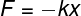Look at the next figure, in which we have a spring in its original shape, then an external force (F ext ) stretches it, causing it to exert an elastic force in the opposite direction:

The amount of energy that was expended to stretch this spring corresponds to the work that had to be done on it. Observing Hooke’s law formula, the greater the elongation of the spring , the greater the elastic force it exerts. Therefore, in order to calculate the work done by the elastic force, we will graph the force versus distance:

F el — Elastic force

To calculate the work that was done in the graph above, it is necessary to calculate its area, which is triangular. Note that the base of this triangle has magnitude x, while its height is determined by the magnitude of the elastic force (F and l = kx). Check the calculation: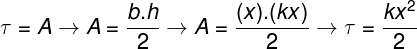According to this calculation, the work required to deform the spring is given by the product of the spring constant (k) by the square of the deformation divided by 2. This amount of work is exactly the amount of energy that is stored by the spring as energy. elastic potential.

## Exercises solved

Question 1) A spring with a spring constant of 20 N/m is stretched and its length, which was initially 20 cm, becomes 50 cm. What is the magnitude of elastic potential energy stored in this spring?

a) 30 J

b) 200 J

c) 0.9 J

d) 50 J

e) 250 J

Template: Letter C

Resolution:

The elastic potential energy can be calculated using this formula:However, to use it, we need to check that all the units provided by the exercise are compatible. In this way, it can be seen that the initial and final sizes of the spring are given in the unit of centimeters (cm), so we need this to be expressed in meters. The strain x is the difference between the final and initial lengths, that is, it is equivalent to 30 cm (0.3 m). Check the calculation: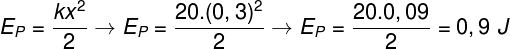Question 2) A spring is compressed by a force of 200 N and its length is reduced by 50 cm. What is the elastic potential energy acquired by the spring?

a) 50 000 J

b) 25,000 J

c) 500 J

d) 50 J

e) 1 200 J

Template: Letter D

Resolution:

The exercise asks us to find the elastic potential energy, however, it does not tell us what the spring constant of the spring is worth so that we can calculate it. For this, we will have to use Hooke’s law and then calculate the elastic potential energy, check: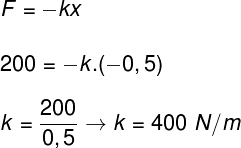After knowing the magnitude of the spring constant, we can calculate its elastic potential energy: